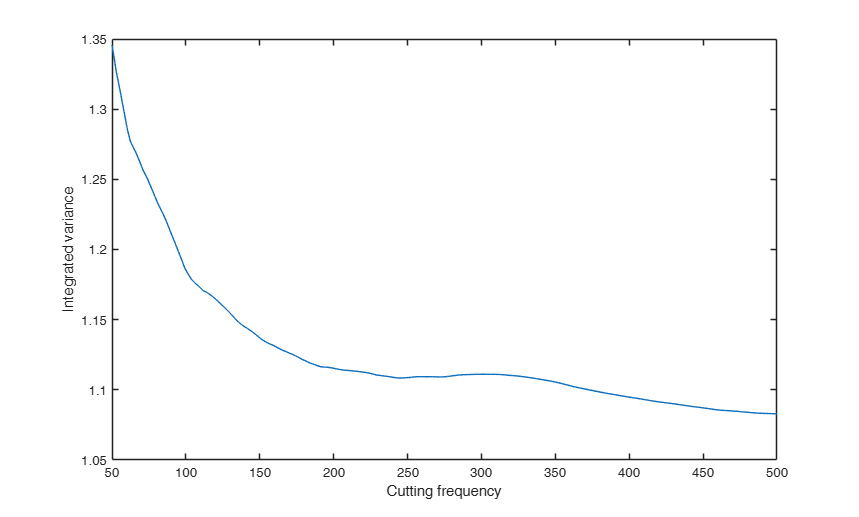FE_int_vol_Fejer

FE_int_vol_Fejer computes the integrated variance from a diffusion process via the Fourier estimator using Fejer kernel

Syntax

• ivar=FE_int_vol_Fejer(x,t)example
• ivar=FE_int_vol_Fejer(x,t,Name,Value)example

Description

FE_int_vol_Fejer computes the integrated variance of univariate timeseries data from a diffusion process by the Fourier estimator with Fejer kernel

ivar =FE_int_vol_Fejer(x, t) Example of call of FE_int_vol_Fejer with just two input arguments.

ivar =FE_int_vol_Fejer(x, t, Name, Value) FE_int_vol_Fejer called with optional input argument N.

Examples

expand allExample of call of FE_int_vol_Fejer with just two input arguments.

The following example calculates the integrated variance from a vector x of discrete observations of a univariate diffusion process

n=1000;
dt=1/n; t=0:dt:1;
x=randn(n,1)*sqrt(dt); x=[0;cumsum(x)];
ivar=FE_int_vol_Fejer(x,t);
disp(['The value of the integrated variance is: ' num2str(ivar)])
The value of the integrated variance is: 1.0678FE_int_vol_Fejer called with optional input argument N.

Analysis of the change of the integrated variance estimates of a univariate diffusion process as a function of the different values of the cutting frequency.

n=1000;
dt=1/n; t=0:dt:1;
x=randn(n,1)*sqrt(dt); x=[0;cumsum(x)];
cuttingfreq=(50:500)';
l=length(cuttingfreq);
Ivar=zeros(l,1);
for i=1:l
ivar=FE_int_vol_Fejer(x,t,'N',cuttingfreq(i));
Ivar(i)=ivar;
end
plot(cuttingfreq,Ivar)
xlabel('Cutting frequency')
ylabel('Integrated variance')Input Arguments

x — Observation values. Vector.

Row or column vector containing the observed values.

Data Types: single| double

t — Observation times. Vector.

Row or column vector with the same length of x containing the observation times

Data Types: single| double

Name-Value Pair Arguments

Specify optional comma-separated pairs of Name,Value arguments. Name is the argument name and Value is the corresponding value. Name must appear inside single quotes (' '). You can specify several name and value pair arguments in any order as Name1,Value1,...,NameN,ValueN.

Example: 'N',500

N —cutting frequency.scalar.

If N is not specified, it is set equal to (length(x)-1)/2.

Example: 'N',500

Data Types: single | double

Output Arguments

ivar —integrated variance. Scalar

Value of the integrated variance.

We assume our timeseries data are discrete observations from a diffusion process $x$ following the Ito stochastic differential equation $$dx(t)= \sigma(t) \ dW(t) + b(t) \ dt,$$ where $W$ is a Brownian motion on a filtered probability space. Let $\sigma$ and $b$ be random processes, adapted to the Brownian filtration.

See the Reference for further mathematical details.

The integrated variance of the process over the time interval $[0,T]$ is defined as $$\int_0^T \sigma^2(t) dt.$$ For any positive integer $n$, let ${\cal S}_{n}:=\{ 0=t_{0}\leq \cdots \leq t_{n}=T \}$ be the observation times. Moreover, let $\delta_i(x):= x(t_{i+1})-x(t_i)$ be the increments of $x$.

The Fejer-Fourier estimator of the integrated variance over $[0,T]$, is defined as $$\widehat\sigma^{2}_{n,N}:= {T^2 \over {N+1}}\sum_{|s|\leq N} \left( 1- {{|s|}\over {N}} \right)c_s(dx_n) c_{-s}(dx_n),$$ where for any integer $k$, $|k|\leq N$, the discretized Fourier coefficients of the increments are $$c_k(dx_{n}):= {1\over {T}} \sum_{i=0}^{n-1} e^{-{\rm i} {{2\pi}\over {T}} kt_i}\delta_i(x).$$ The cutting frequency $N$ is a scalar integer. If not specified, $N$ is set equal to $n/2$ (Nyquist frequency).

Mancino, M.E., Recchioni, M.C., Sanfelici, S. (2017), Fourier-Malliavin Volatility Estimation. Theory and Practice, "Springer Briefs in Quantitative Finance", Springer.